# Summary table of probability terms

## Table

Term Notation Type Definition Notes
Reals$\mathbf R$
Borel subsets of the reals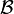$\mathcal B$
A Borel set$B$$\mathcal B$
Sample space$\Omega$
Outcome$\omega$$\Omega$
Events or measurable sets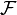$\mathcal F$
Probability measure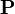$\mathbf P$ or$\Pr$ or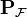$\mathbf P_{\mathcal F}$$\mathcal F \to [0,1]$
Probability triple or probability space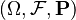$(\Omega, \mathcal F, \mathbf P)$
Distribution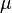$\mu$ or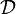$\mathcal D$ or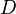$D$ or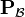$\mathbf P_{\mathcal B}$ or$\mathcal L(X)$ or$\mathbf P X^{-1}$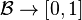$\mathcal B \to \mathbf [0,1]$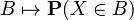$B \mapsto \mathbf P(X \in B)$
Induced probability space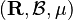$(\mathbf R, \mathcal B, \mu)$
Cumulative distribution function or CDF$F_X$$\mathbf R \to [0,1]$
Probability density function or PDF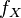$f_X$$\mathbf R \to [0,\infty)$
Random variable$X$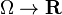$\Omega \to \mathbf R$
Preimage of random variable$X^{-1}$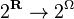$2^{\mathbf R} \to 2^{\Omega}$ but all we need is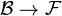$\mathcal B \to \mathcal F$
Indicator of$A$$1_A$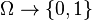$\Omega \to \{0,1\}$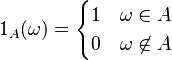$1_A(\omega) = \begin{cases}1 & \omega\in A \\ 0 & \omega \not\in A\end{cases}$
Expectation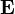$\mathbf E$ or$\mathrm E$$(\Omega \to \mathbf R) \to \mathbf R$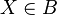$X \in B$$\mathcal F$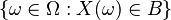$\{\omega \in \Omega : X(\omega) \in B\}$$X=x$$\mathcal F$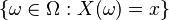$\{\omega \in \Omega : X(\omega) = x\}$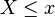$X\leq x$$\mathcal F$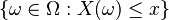$\{\omega \in \Omega : X(\omega) \leq x\}$
Function of a random variable, where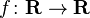$f\colon \mathbf R \to \mathbf R$$f(X)$$\Omega \to \mathbf R$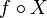$f\circ X$
Expected value of$X$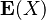$\mathbf E(X)$$\mathbf R$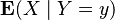$\mathbf E(X\mid Y=y)$$\mathbf R$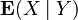$\mathbf E(X\mid Y)$$\Omega \to \mathbf R$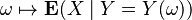$\omega \mapsto \mathbf E(X\mid Y=Y(\omega))$?
Utility function$u$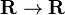$\mathbf R \to \mathbf R$ I think this is what the type must be, based on how it's used. But we usually think of the utility function as assigning numbers to outcomes; but if that is so, it must be a random variable! What's up with that?
Expected utility of$X$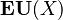$\mathbf{EU}(X)$$\mathbf R$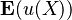$\mathbf E(u(X))$$u\circ X$ is indeed a random variable, so the type check passes.

All the utility stuff isn't really related to machine learning. It's more related to the decision theory stuff I'm learning. I'm putting it here for now for convenience but might move it later.

TODO add "probability distribution over S" and "probability distribution on A" 

Li and Vitanyi (An Introduction to Kolmogorov Complexity and Its Applications, p. 19) calls the probability measure on$\mathcal F$ a probability distribution over S (the sample space).

TODO: add probability mass function (defined only for discrete random variables)

## Dependencies

Let$(\Omega, \mathcal F, \mathbf P)$ be a probability space.

• Given a random variable$X$, we can compute its distribution$\mu$. How? Just let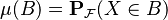$\mu(B) = \mathbf P_{\mathcal F}(X \in B)$
• Given a random variable, we can compute the probability density function. How?
• Given a random variable, we can compute the cumulative distribution function. How?
• Given a distribution, we can retrieve a random variable. But this random variable is not unique? This is why we can say stuff like "let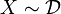$X\sim \mathcal D$".
• Given a distribution$\mu$, we can compute its density function. How? Just find the derivative of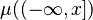$\mu((-\infty,x])$. (?)
• Given a cumulative distribution function, we can compute the random variable. (Right?)
• Given a probability density function, can we get everything else? Don't we just have to integrate to get the cdf, which gets us the random variable and the distribution?
• Given a cumulative distribution function, how do we get the distribution? We have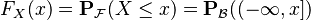$F_X(x) = \mathbf P_{\mathcal F}(X\leq x) = \mathbf P_{\mathcal B}((-\infty,x])$, which gets us some of what the distribution$\mathbf P_{\mathcal B}$ maps to, but$\mathcal B$ is bigger than this. What do we do about the other values we need to map? We can compute intervals like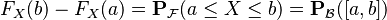$F_X(b) - F_X(a) = \mathbf P_{\mathcal F}(a \leq X\leq b) = \mathbf P_{\mathcal B}([a,b])$. And we can apparently do the same for unions and limiting operations.

## Philosophical details about the sample space

Given a random variable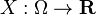$X : \Omega \to \mathbf R$ and any reasonable predicate$P$ about$X$, we can replace$P(X)$ with its extension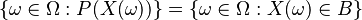$\{\omega \in \Omega : P(X(\omega))\} = \{\omega \in \Omega : X(\omega) \in B\}$ for some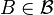$B \in \mathcal B$. And from then on, we can write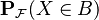$\mathbf P_{\mathcal F}(X\in B)$ as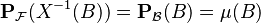$\mathbf P_{\mathcal F}(X^{-1}(B)) = \mathbf P_{\mathcal B}(B) = \mu(B)$. In other words, we can just work with Borel sets of the reals (measuring them with the distribution) rather than the original events (measuring them with the original probability measure). Where did$X$ go?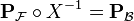$\mathbf P_{\mathcal F} \circ X^{-1} = \mathbf P_{\mathcal B}$, so you can write$\mathbf P_{\mathcal B}$ using$X$. But once you already have$\mathbf P_{\mathcal B}$, you don't need to know what$X$ is.